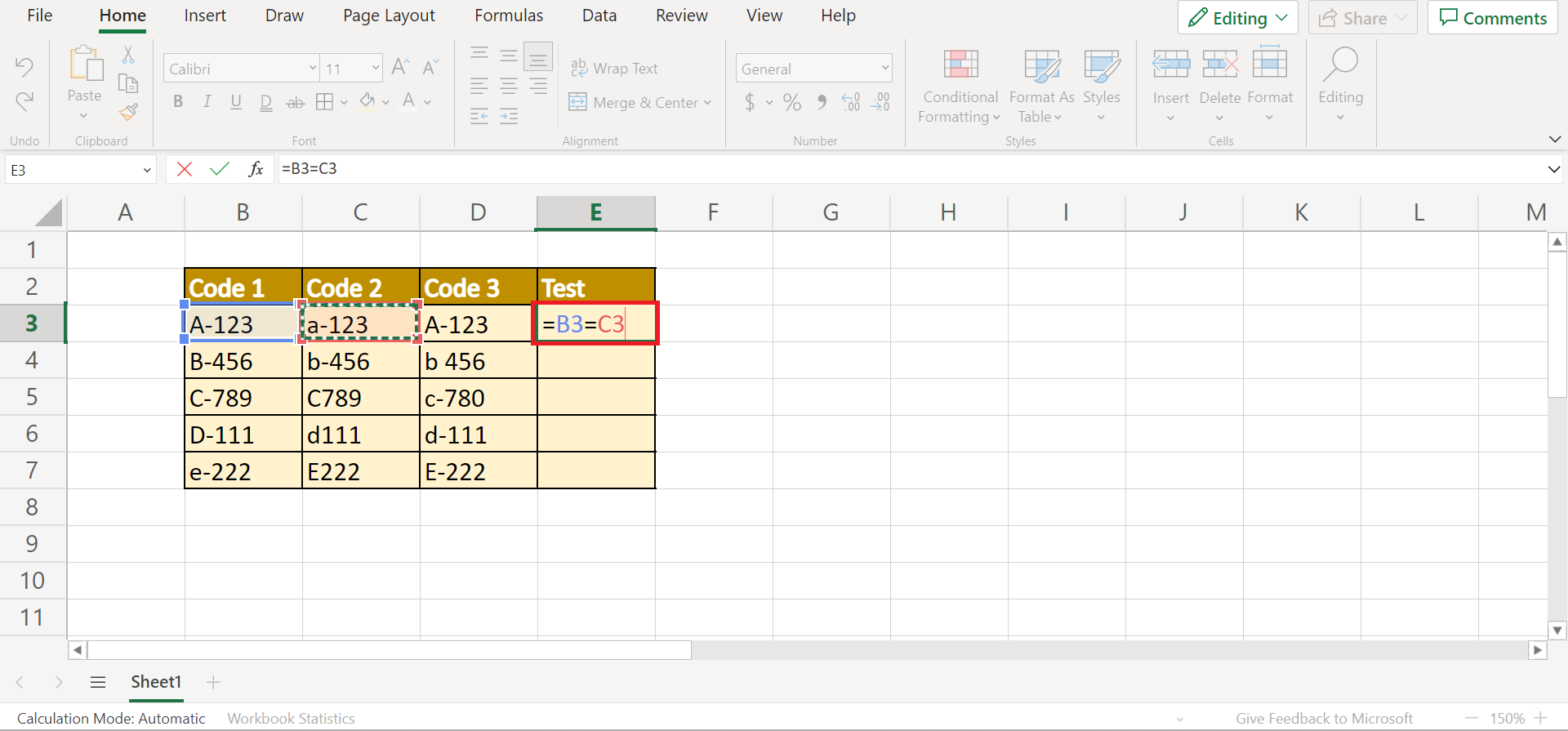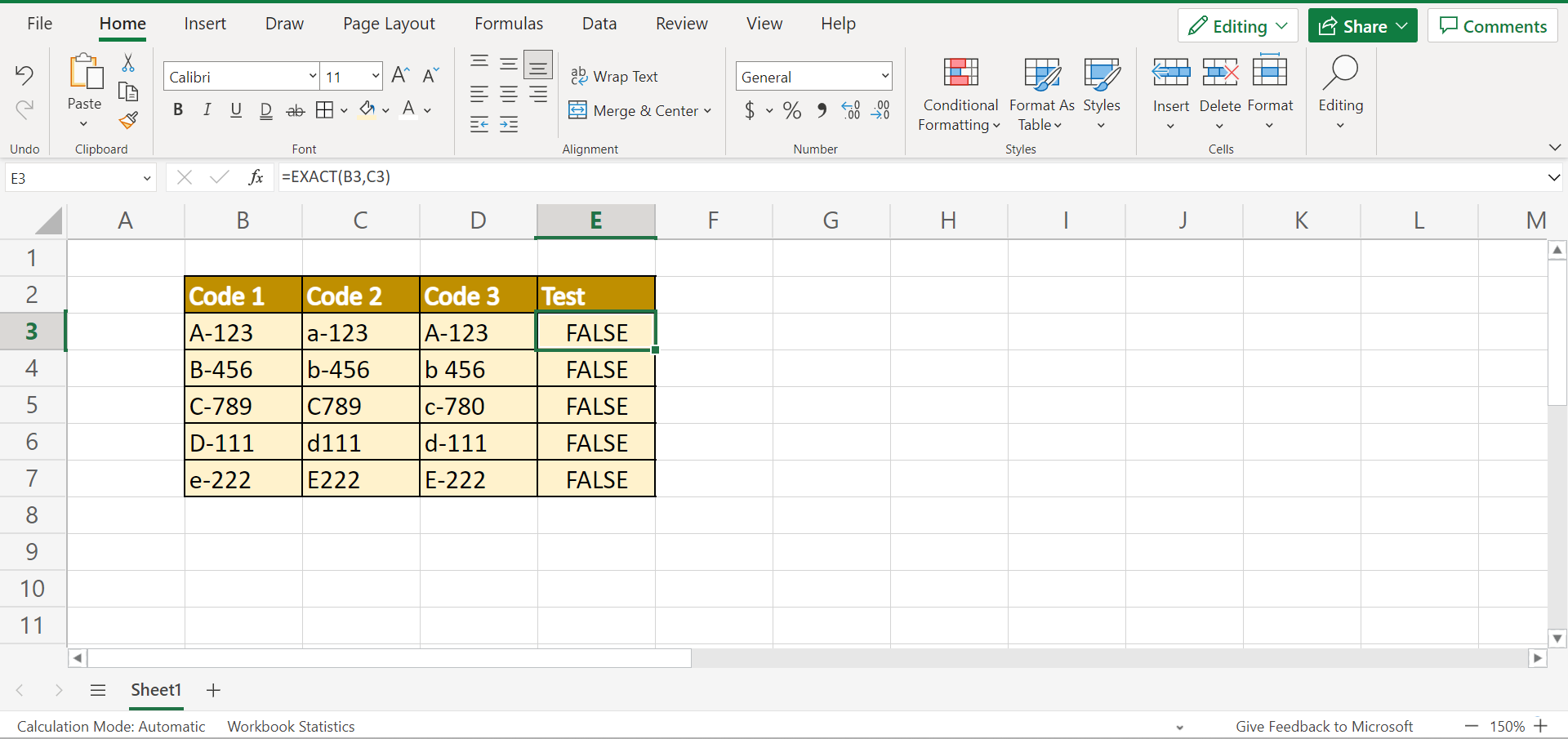# How to Check if Two Cells Match or Multiple Cells are Equal in Excel

This guide will discuss how to check if two cells match or if multiple cells are equal in Excel

Excel is a popular tool for calculations, analyzing, organizing, and reporting data. And one of the things we can do in Excel is to check whether two cells match or multiple cells are equal. Aside from simply looking at the values, we can use different functions to compare the values accurately.

Furthermore, we may need to check if two cells or multiple cells match or is equal before performing a formula or calculation. So having an idea whether values match or are equal would lessen the chances of an error returning after performing a formula.

So this guide will discuss 5 simple and efficient methods to check whether two cells match or if multiple cells are equal in Excel.

Let’s take a sample scenario wherein we must check if the cells match.

Suppose you were tasked to compare the values between different codes. And you had to figure out whether the codes matched or were equal to one another. But, you were worried about making a mistake if you only looked at the values individually.

For accuracy and efficiency, you opted to use a function in Excel to check if the codes match or were equal. And this made the entire process faster and easier.

Great! Now let’s move on and discuss the five methods we can use to check if two cells match or if multiple cells are equal in Excel.

## Using the Equal Sign

The first method, which is also the easiest method, is to use the equal sign to compare whether two cells match or are equal. To perform this method, simply follow the steps below:

1. Firstly, we must select a cell to input the results. In this case, we will type in cell E3. Then, we simply need to type in an equal sign and select the two cells to compare. So the entire formula would be “=B3=C3”. Lastly, press the Enter key to return the results.2. Secondly, we can drag down the formula to copy it to the rest of the column. If we get a result of TRUE, it means the two cells match. If we get a FALSE result, the two cells do not match.3. And tada! We have successfully compared the two cells and checked whether they match or not.## Using the TRIM function

Next, we can use the `TRIM` function to check if the two cells match. But, the difference between using this function and just the equal sign is that the `TRIM` function will remove the leading spaces, extra spaces, or trailing spaces between the values.

If we are not too concerned about the spaces to decide whether two cells match, this is the perfect method to use. To perform this method, follow the steps below.

1. Firstly, we must select a cell to input the formula and return the result. In this case, we will choose cell. In cell E3, type in the formula “=TRIM(B3)=TRIM(C3)”. Then, press the Enter key to return the result.2. Secondly, we can simply drag down the formula to copy it instead of typing the formula again and again.3. And tada! We have checked whether the two cells match without the extra spaces between the values.## Using the COUNTIF function

Thirdly, we can utilize the `COUNTIF` function to check if multiple cells are equal. So the `COUNTIF` function will count the number of cells within a selected range that meet the given condition. Furthermore, the `COUNTIF` function only has two arguments. Both of which are required arguments.

Firstly, we have the range, which refers to the selected range we want to count from. Then, we have the criteria, which refer to the condition in the form of a number, text, or expression that will define what cells will be counted.

To use the `COUNTIF` function, let’s follow the steps below:

1. Firstly, we need to select a cell to input the results. In this case, we will input an equal sign and the `COUNTIF` function in cell E3. Then, we will select the cells we want to compare. So our entire formula would be “=COUNTIF(B3:D3,B3)=3”. Lastly, press the Enter key to get the results.2. Secondly, copy the formula by dragging it down to the rest of the column.3. And tada! We have checked whether multiple cells are equal using the `COUTNIF` function.## Using the AND function

So our fourth method is by using the `AND` function in Excel. The `AND` function will check all the values one by one and return a boolean result. If the AND function returns TRUE, all the values in the cells are the same. If the `AND` function returns a FALSE, all the values in the cells are not the same.

Furthermore, the `AND` function has one required argument and one optional argument. Firstly, we have logical1, which is a required argument referring to the condition we want to test. And this can be arrays, cell references, or logical values,

Next, we have the optional argument logical2, which is simply another condition we want to test.

To use this method, follow the steps below:

1. Firstly, we must select a cell to input the formula. In this case, we will choose cell E3. Then, type in the formula “=AND(B3=C3:D3)”. Lastly, press the Enter key to return the results.2. Secondly, we will pull down the fill handle to copy the formula to the rest of the cells.3. And tada! We have successfully used the `AND` function to check whether the multiple cells are equal.## Using the EXACT function

Lastly, we can use the `EXACT` function to compare if two cells match exactly. So the `EXACT` function will check for an exact match between text strings, including lower and upper case. And this function will return a TRUE or FALSE.

Additionally, the `EXACT` function only has two required arguments. First, we have text1, the first text string we want to compare. Secondly, we have text2, the second text string we want to compare to the first selected text.

Now let’s learn the steps of how to use the `EXACT` function.

1. Firstly, we need to select a cell to input the function in. In this case, we will type the formula in cell E3. So our entire formula would be “=EXACT(B3,C3)”. Then, press the Enter key to return the results.2. Secondly, drag down to copy the formula.3. And tada! We have successfully used the `EXACT` function to check if two cells match.And that’s pretty much it! We have discussed five simple and efficient methods to check if two cells match or if multiple cells are equal in Excel. Now you can simply choose any method to use in your work.

Are you interested in learning more about what Excel can do? You can now use the `EXACT` function and the various other Microsoft Excel formulas available to create great worksheets that work for you. Make sure to subscribe to our newsletter to be the first to know about the latest guides and tutorials from us.Our goal this year is to create lots of rich, bite-sized tutorials for Google Sheets users like you. If you liked this one, you'll love what we are working on! Readers receive ✨ early access ✨ to new content.

##### You May Also Like## How to Rank Non-Continuous Range in Excel

This guide will explain how you can use the RANK function to rank a non-continuous range in Excel.…## How to Group Columns in Excel

This guide will explain how to group columns in Excel using the built-in Group feature. Users can take…## How to Use ANOVA Two Factor Without Replication in Excel

This guide will explain how to create a two-factor ANOVA analysis without replication in Excel. This type of…## How to Stack Multiple Columns into One Column in Excel

The CONCAT and TEXTJOIN functions are useful when you need to combine multiple columns of text values into…